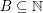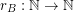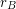Importance: Outstanding ✭✭✭✭
 Author(s): Erdos, Paul Turan, Paul
 Subject: Number Theory » Additive Number Theory
 Prize: \$500 (Erdos - Graham [EG])
 Posted by: mdevos on: June 8th, 2007

Let. The representation functionforis given by the rule. We callan additive basis ifis never.

Conjecture   Ifis an additive basis, thenis unbounded.

This famous conjecture seems intuitively likely, but to date, there has been relatively little progress on it despite considerable attention. Two positive results are a theorem of Dirac [D] which shows thatcannot be constant from some point on, and a theorem of Borwein, Choi, and Chu [BCC] which shows thatcannot be bounded above by.

On the other hand, if we consider the related problem for subsets of integers instead of natural numbers, Nathanson [N] has shown that the conjecture does not hold.

## Bibliography

[BCC] P. Borwein, S. Choi, and F. Chu, An old conjecture of Erdos-Turan on additive bases, Mathematics of Computation. Volume 75, Number 253, Pages 475–484.

[D] G. A. Dirac, Note on a problem in additive number theory, J. London Math. Soc. 26 (1951), 312–313.

[EG] P. Erdos and R. L. Graham, Old and new problems and results in combinatorial number theory: van der Waerden’s theorem and related topics, Enseign. Math. (2) 25 (1979), no. 3-4, 325–344 (1980). MathSciNet

*[ET] P. Erdos and P. Turan, On a problem of Sidon in additive number theory, and on some related problems, J. London Math. Soc. 16 (1941), 212–215. MathSciNet

[N] Melvyn B. Nathanson, Unique representation bases for the integers, Acta Arith. 108 (2003), no. 1, 1–8. MathSciNet

* indicates original appearance(s) of problem.